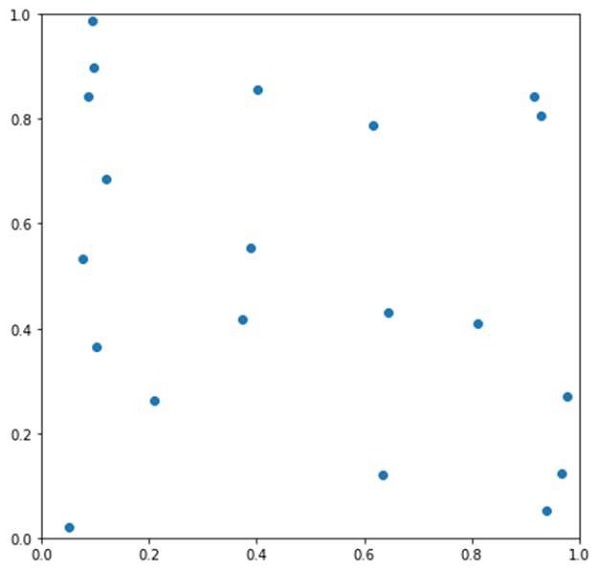# Generating a movie from Python without saving individual frames to files

Using the FuncAnimation method, we can create a film. We will create a user-defined method, update, to keep on changing the position of particles and at the end, the method would return the scatter instance.

## Steps

• Get the particles initial position, velocity, force, and size.

• Create a new figure, or activate an existing figure with figsize = (7, 7).

• Add an axes to the current figure and make it the current axes, with xlim and ylim.

• Plot the scatter for initial position of the particles.

• Makes an animation by repeatedly calling a function *func*. We can pass a user-defined method that helps to change the position of particles, into FuncAnimation class.

• Show the figure using plt.show().

## Example

import matplotlib.pyplot as plt
from matplotlib.animation import FuncAnimation
import numpy as np

dt = 0.005
n = 20
L = 1
particles = np.zeros(n, dtype=[("position", float, 2),
("velocity", float, 2),
("force", float, 2),
("size", float, 1)])

particles["position"] = np.random.uniform(0, L, (n, 2));
particles["velocity"] = np.zeros((n, 2));
particles["size"] = 0.5 * np.ones(n);

fig = plt.figure(figsize=(7, 7))
ax = plt.axes(xlim=(0, L), ylim=(0, L))
scatter = ax.scatter(particles["position"][:, 0], particles["position"][:, 1])

def update(frame_number):
particles["force"] = np.random.uniform(-2, 2., (n, 2));
particles["velocity"] = particles["velocity"] + particles["force"] * dt
particles["position"] = particles["position"] + particles["velocity"] * dt

particles["position"] = particles["position"] % L
scatter.set_offsets(particles["position"])
return scatter,

anim = FuncAnimation(fig, update, interval=10)
plt.show()

## Output